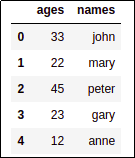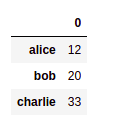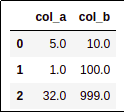Pandas Dataframe Examples: Create and Append data

# Pandas Dataframe Examples: Create and Append data

Last updated:

Pandas version used: 1.0.3

There are many ways to build and initialize a pandas DataFrame. Here are some of the most common ones:

All examples can be found on this notebook

## Create from lists

Where each list represents one column.

import pandas as pd

names = ['john','mary','peter','gary','anne']
ages = [33,22,45,23,12]

df = pd.DataFrame({
'names':names,
'ages':ages
})
dfProbably the most straightforward
way to build dataframes

## Create from dicts

To create a dataframe from a list of dicts use pd.DataFrame.from_records().

import pandas as pd

data_dicts = [
{'name':"john","gender":'male','age':45},
{'name':"mary", 'gender':"female",'age':19},
{'name':"peter",'gender':'male', 'age':34}
]

df = pd.DataFrame.from_records(data_dicts)
dfSince we didn't specify dtypes, they are automatically inferred from the data.

## Create from dict

To create a dataframe from a single dict using keys the index use pd.DataFrame.from_dict(my_dict, orient='index')

import pandas as pd

d = {"alice": 12, "bob": 20, "charlie": 33}

pd.DataFrame.from_dict(d, orient='index')SOURCE DICT: just a simple dictYou can set the name of the column
if you want, passing columns=['age'] to from_dict

## Create empty Dataframe, append rows

Use append() with ignore_index=True.

import pandas as pd

# if you wish, you can set column names and dtypes here
df = pd.DataFrame()

# must reassign since the append method does not work in place
df = df.append({'col_a':5,'col_b':10}, ignore_index=True)
df = df.append({'col_a':1,'col_b':100}, ignore_index=True)
df = df.append({'col_a':32,'col_b':999}, ignore_index=True)

dfSince ignore_index is set, indices will start at 0